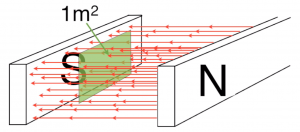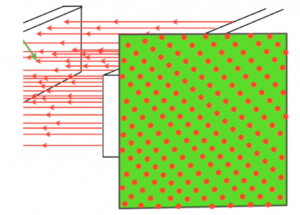### Magnetic flux

We indirectly use Faraday's Law to define magnetic flux. Imagine a wire cutting down through this magnetic field. The EMF produced depends upon how fast we cut through the field. If we move the wire faster, if the magnetic field is stronger or if there are more coils of wire then the EMF will be larger.

So the EMF at any instant is equal to the rate at which we are cutting the flux, the mathematical equation is this:

So we use this to define magnetic flux, which is measured in webers (Wb) that is:  A flux of one weber cut through by a wire each second induces an EMF of one volt.

### Magnetic flux density

Think of this improbably large magnet with a sheet of green paper with an area one square metre perpendicular to the field.If the total flux cutting through one square metre of the field is one weber, then the flux density is one Tesla (T). So a tesla is webers per square metre.

### Magnetic flux and magnetic flux density video lesson

Other useful pages on magnetism and electromagnetism  are: